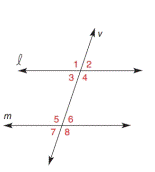Chapter 2.3, Problem 4EElementary Geometry For College St...

7th Edition
Alexander + 2 others
ISBN: 9781337614085

Solutions

Chapter
SectionElementary Geometry For College St...

7th Edition
Alexander + 2 others
ISBN: 9781337614085
Textbook Problem

In Exercises 1 to 6 , l and m are cut by transversal v . On the basis of the information given, determine whether l must be parallel to m . m ∠ 1 = 106 ° and m ∠ 4 = 106 °To determine

To check:

The given statement.

Explanation

Given:

and m are cut by transversal v.

m1=106° and m4=106°

Approach:

Vertically opposite angles are congruent to each other.

Calculation:

The given statement is,

and m are cut by transversal v

Still sussing out bartleby?

Check out a sample textbook solution.

See a sample solution

The Solution to Your Study Problems

Bartleby provides explanations to thousands of textbook problems written by our experts, many with advanced degrees!

Get Started

Find limh01h22+h1+t3dt

Single Variable Calculus: Early Transcendentals, Volume I

Find f. f(x) = 5x4 3x2 + 4, f(1) = 2

Single Variable Calculus: Early Transcendentals

For f(x)=x2+sinhx, f(x) = a) 2x+coshx2x2+sinhx b) x+coshxx2+sinhx c) 122x+coshx d) xcoshxx2+sinhx

Study Guide for Stewart's Single Variable Calculus: Early Transcendentals, 8th

Evaluate 6 8 24 30

Study Guide for Stewart's Multivariable Calculus, 8th